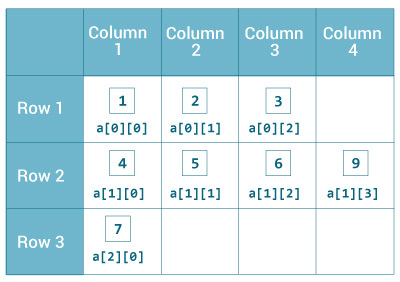In this article, we will find something out about array in Java Core. This is a fundamental knowldege that we need to know when working with Java.

Let’s get started.

## 1D Array

### Declaration an array

``````data_type[] name_variable;
``````
• `data_type`: primitive data type such as `int`, `char`, `Double`, `byte` or object.
• `name_variable`: identifier.

For example:

``````int[] values;
Double[] data;
``````

### Allocate memory for an 1D array

``````values = new int;
data = new Double;
``````

Once the length of an array is defined, it cannot changed in the program.

The index of an array starts from 0. So, the first element is `name_variable`, and the last element is `name_variable[n - 1]`.

The default initial value of elements of an array is `0` for `numeric types` and `false` for `boolean`.

### Initialize an array

``````int[] values = {1, 2, 3, 4, 5};
``````

## Multidimensional Array

### Declaration an array

``````int[][] values;
String[][][] data;
``````

Multidimensional array is an array that contains other arrays.### Allocate memory for an nD array

``````values = new int;
data = new String;
``````

We can ignore the number of columns

``````String[][] data = new String[];
``````

but to be able to fill it, you will have to initialize each row separately:

``````String[][] data = new String[];
data = new String;
data = new String;
``````

### Initialize an array

``````int[][] values = {
{1, 2, 3},
{4, 5, 6},
{7}
};

int[][][] test = {
{
{1, -2, 3},
{2, 3, 4}
},
{
{-4, -5, 6, 9},
{1},
{2, 3}
}
};
``````

Refer:

https://www.programiz.com/java-programming/multidimensional-array

http://grails.asia/two-dimensional-string-array-in-java